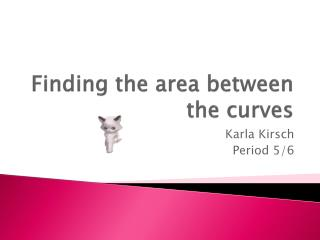DownloadDownload PresentationFinding the area between the curves

# Finding the area between the curves

Télécharger la présentation## Finding the area between the curves

- - - - - - - - - - - - - - - - - - - - - - - - - - - E N D - - - - - - - - - - - - - - - - - - - - - - - - - - -
##### Presentation Transcript

1. Finding the area between the curves Karla Kirsch Period 5/6

2. THE QUESTION

3. a) Find the area of R. • In order to find the area of R, you need to… • Figure out which equation is the top and which one is the bottom • Find out the interval of the figure • Integrate the function using top minus bottom • Plug into the calculator and solve. Top function y=6 Bottom function y=4ln(3-x)

4. Create the integral and plug it into the calculator Answer: R = 6.817

5. b) Find the volume of the solid generated when R is revolved about the horizontal line y=8 • In order to find the volume of a solid you must use the equation: : • You take whatever line the solid is being revolved around, and subtract each equation from it • Plug this into the equation and plug into the calculator

6. Plug the values into the equation and solve on the calculator • R(x)= (8-4ln(3-x)) r(x)= (8-6) Answer : V= 168.179

7. c) The region R is the base of a solid. For this solid, each cross section perpendicular to the x-axis is a square. Find the volume of the solid. • When using cross sections, you must first identify the shape that is described in the problem; which is a square for this question. • Once you identify the shape, figure out the formula for the area of that shape. • Volume of the solid= Area between the curves, plugged into the formula for area of the cross section • V = ∫(A)² dx

8. Plug values into the equation of volume • R=(6-4ln(3-x)) ∙∘∙∘∙ Area of square=(x)² Area of a square equation Area between the curves

9. Plug the equation into the calculator and solve Answer : V=26.266

10. CITATIONS • http://apcentral.collegeboard.com/apc/public/repository/ap10_calculus_ab_form_b_q1.pdf • http://www.animatedgif.net/ • http://mszhao.com/

11. THE END ☺♥